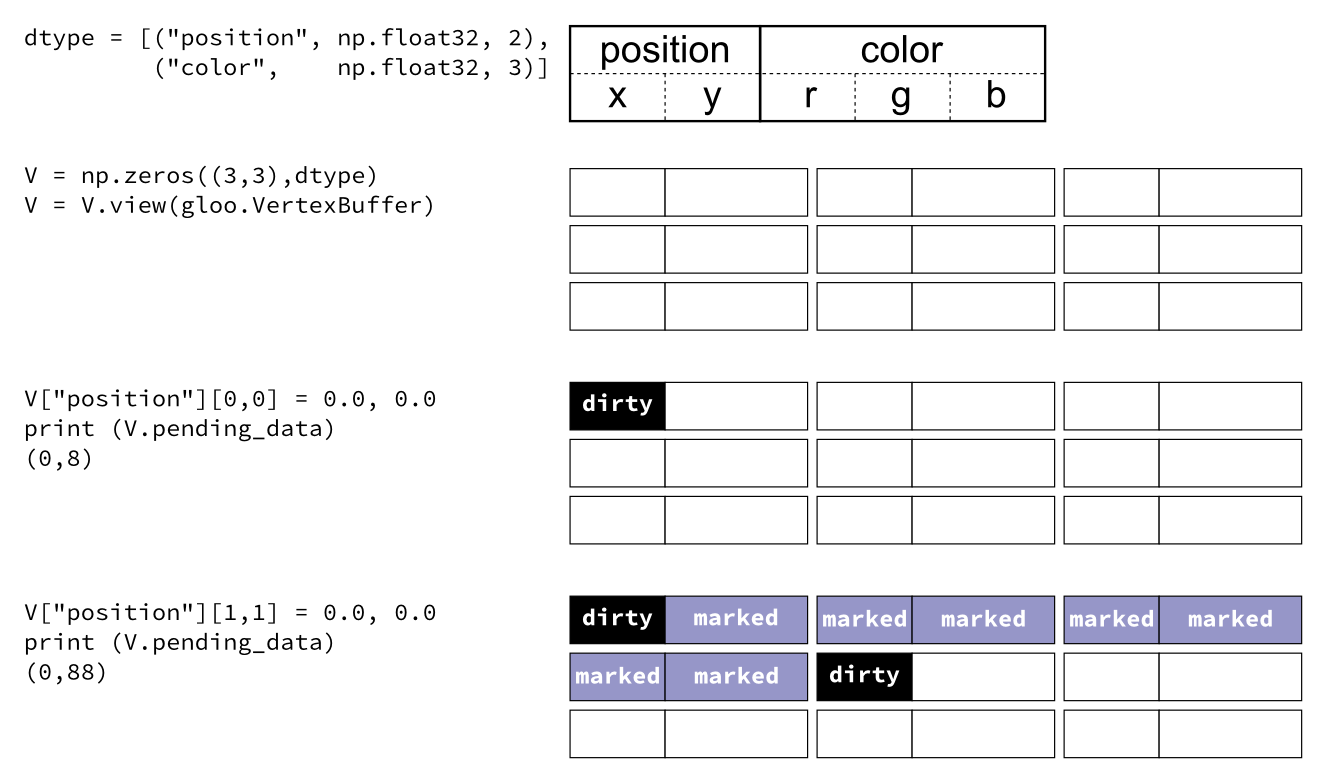# Numpy integration¶

Glumpy is based on a tight and seamless integration with numpy arrays. This means you can manipulate GPU data as you would with regular numpy arrays and glumpy will take care of the rest. But an example is worth a thousand words:

```from glumpy import gloo

dtype = [("position", np.float32, 2),  # x,y
("color",    np.float32, 3)]  # r,g,b
V = np.zeros((3,3),dtype).view(gloo.VertexBuffer)
V["position"][0,0] = 0.0, 0.0
V["position"][1,1] = 0.0, 0.0
```

V is a `VertexBuffer` which is both a `GPUData` and a numpy array. When V is modified, glumpy takes care of computing the smallest contiguous block of dirty memory since it was last uploaded to GPU memory. When this buffer is to be used on the GPU, glumpy takes care of uploading the “dirty” area at the very last moment. This means that if you never use V, nothing will be ever uploaded to the GPU! In the case above, the last computed “dirty” area is made of 88 bytes starting at offset 0 as illustrated below:Note

When a buffer is created, it is marked as totally dirty, but for the sake of illustration, just pretend this is not the case here.

Glumpy will thus end up uploading 88 bytes while only 16 bytes have been actually modified. You might wonder if this optimal. Actually, most of the time it is. Uploading some data to a buffer requires a lot of operations on the GL side and each call has a fixe cost. For example, consider the gloo-quad.py example from the examples directory. If you start this program with the `--debug` flag, you’ll see what is really happening in the shadow to just display this simple quad:

```\$ python gloo-quad.py -f 1 --debug
[i] HiDPI detected, fixing window size
[i] Using GLFW (GL 2.1)
[i] Running at 1 frames/second
GPU: Creating program
GPU: Activating program (id=1)
GPU: Activating buffer (id=5)
GPU: Creating buffer (id=5)
GPU: Deactivating buffer (id=5)
GPU: Activating buffer (id=5)
GPU: Updating position
GPU: Deactivating buffer (id=5)
GPU: Deactivating program (id=1)
GPU: Activating program (id=1)
GPU: Activating buffer (id=5)
GPU: Deactivating buffer (id=5)
GPU: Deactivating program (id=1)
GPU: Activating program (id=1)
GPU: Activating buffer (id=5)
GPU: Deactivating buffer (id=5)
GPU: Deactivating program (id=1)
```

The glumpy philosophy is thus to minimize GL calls and to try to merge any buffer update operations into a single call sequence, even if it is suboptimal in some cases.

Note

Note that you can nevertheless optimize the code above by splitting the buffer into one vertex buffer for colors and one vertex buffer for positions. If colors are rather static and positions rather dynamic, you’ll save the cost of uploading colors each time you change some positions.